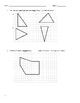# Open Up Resources Unit 1 End-of-Unit Practice Problems - Area and Surface AreaSubject
Resource Type
File Type

PDF

(322 KB|4 pages)
Product Rating
Standards
• Product Description
• StandardsNEW

A set of 11 practice problems aimed to help students study for the end-of-unit assessment for unit 1: Area and Surface Area.

Questions focus on:

- Identifying the base and height of parallelograms and triangles.

- Finding the area of parallelograms and triangles.

- Finding the area of an irregular polygon using what students know about area of parallelograms and triangles.

- Identifying (naming) and finding the surface area of polyhedron and nets of polyhedron.

- Finding the area and volume of a cube.

- Exponents.

Evaluate expressions at specific values of their variables. Include expressions that arise from formulas used in real-world problems. Perform arithmetic operations, including those involving whole-number exponents, in the conventional order when there are no parentheses to specify a particular order (Order of Operations). For example, use the formulas V = s³ and A = 6 s² to find the volume and surface area of a cube with sides of length s = 1/2.
Write and evaluate numerical expressions involving whole-number exponents.
Represent three-dimensional figures using nets made up of rectangles and triangles, and use the nets to find the surface area of these figures. Apply these techniques in the context of solving real-world and mathematical problems.
Find the volume of a right rectangular prism with fractional edge lengths by packing it with unit cubes of the appropriate unit fraction edge lengths, and show that the volume is the same as would be found by multiplying the edge lengths of the prism. Apply the formulas 𝘝 = 𝘭 𝘸 𝘩 and 𝘝 = 𝘣 𝘩 to find volumes of right rectangular prisms with fractional edge lengths in the context of solving real-world and mathematical problems.
Find the area of right triangles, other triangles, special quadrilaterals, and polygons by composing into rectangles or decomposing into triangles and other shapes; apply these techniques in the context of solving real-world and mathematical problems.
Total Pages
4 pages
Included
Teaching Duration
N/A
Report this Resource to TpT
Reported resources will be reviewed by our team. Report this resource to let us know if this resource violates TpT’s content guidelines.